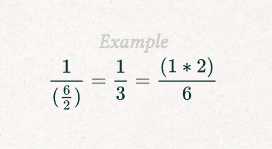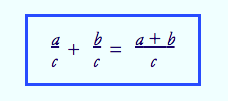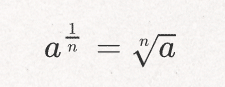"An ounce of algebra is worth of a ton of verbal argument." -John B. S Haldane

The quote from John B. S Haldane mentioned above could not be more accurate. The topic of algebra has the power to divide opinions fiercely: some love analysing algebra while others couldn't hate it more.

In today's article, we will analyse some basic algebra rules, equations, examples, and definitions to make algebraic information more enjoyable to all types of learners.The best Math tutors available5 (96 reviews)
Hamid
\$78
/h1st lesson is free!5 (81 reviews)
Laura
\$50
/h1st lesson is free!5 (15 reviews)
Gurman
\$25
/h1st lesson is free!5 (15 reviews)
Justin
\$30
/h1st lesson is free!5 (75 reviews)
Brendon
\$17
/h1st lesson is free!4.9 (28 reviews)
Oluwatobi
\$15
/h1st lesson is free!4.9 (14 reviews)
Stewart
\$60
/h1st lesson is free!5 (71 reviews)
Alaa
\$50
/h1st lesson is free!5 (96 reviews)
Hamid
\$78
/h1st lesson is free!5 (81 reviews)
Laura
\$50
/h1st lesson is free!5 (15 reviews)
Gurman
\$25
/h1st lesson is free!5 (15 reviews)
Justin
\$30
/h1st lesson is free!5 (75 reviews)
Brendon
\$17
/h1st lesson is free!4.9 (28 reviews)
Oluwatobi
\$15
/h1st lesson is free!4.9 (14 reviews)
Stewart
\$60
/h1st lesson is free!5 (71 reviews)
Alaa
\$50
/h1st lesson is free!

## The Fundamental Rules of AlgebraFrom sports to algebra, there are rules for everything. (Source: pixabay)

Without rules and restrictions, society cannot effectively exist; we need to be told what to do to know our limitations. Therefore, concepts and disciplines that are commonly used in modern-day society need to have regulations for them to function correctly and be understood by humans.

It is essential to state that there are rules for everything in life. From calculating fuel consumption to determining the number of trips made per hour by a waiter, algebra is continuously in use.

Therefore, without further delay, we will consider some of the algebra rules for arithmetic, exponents, and radicals.

### Rules of Algebra Associated with Arithmetic

#### Distributive Property of Multiplication

The distributive property of multiplication is based on the fact that you're multiplying something with a sum of two or more other terms. Your multiplication can be distributed to each one of the different terms linked to the equation. An example of the distributive property of repetition is the following:

y(x + y) = xy + xz

After the necessary order of operations has been developed, the answer or problem has been found.

#### Rules For Adding, Subtracting, Dividing, and Multiplying Fractions

The laws of algebra for arithmetic slightly change when dealing with equations that feature fractions.

For example, if you have the purpose of multiplying a fraction, the numerator of a fraction applies to the fraction as a complete figure. Therefore, if you need to multiply a fraction, times the numerator and the objective is achieved.

The following example will aid those who are more visual when attempting to multiply fractions:

6 (1/3) = ab/c

Dividing a portion is very closely linked to the previously mentioned rule about multiplication. By dividing the denominator of a fraction, it will have the same effect as multiplying the numerator. The following is an example to understand better:A perfect example of the rules of dividing a fraction in algebra. (Source: Algebrarules.com)

The rules of adding and subtracting fractions are the same: the denominators must be identical; just as it is in arithmetic. The numerators must be attached, and their sums must be placed over the common denominator. The following is a great example to understand the basics of adding or subtracting fractions in algebra:The rules of adding and subtracting portions remain the same; only the symbols change. (Source: themathpage.com)

There are plenty of other algebra rules associated with arithmetic that can be analysed; the previously mentioned are only a few that should be mastered from the beginning.

### Algebra Rules for Exponents

If you are reading this article and have forgotten what exponents are, we will refresh your memory. Exponents are a quantity that represents the power to which a number or expression is to be raised, usually expressed as a raised symbol beside the name or phrase in algebra.

Just as there are rules for everything else in algebra, the following are some of the regulations for exponents:

• Zero-Exponent Rule: for example, a0 = 1 means that anything raised to the zero power is 1.
• Power Rule: also known as powers to powers rules, it is essential to state that to raise a power to power you need to multiply the exponents. Other minor regulations associated with the "powers to powers" include the quotient to power rule.
• Product Rule: the product of two powers with the same base is equal to the base raised to the sum of the two exponents. This rule can be effectively used by writing out the exponents as multiplications; this makes the equation appear realistic and accurate.

Find a maths tutor near me here.

### The Distinct Rules of Algebra for Radicals

In the full world of algebra, radical expressions are essential and can be viewed as the polar opposite of exponential expressions since they yield roots.

One typical rule associated with radicals in algebra can be seen as the following:While radicals and exponents are quite distinct, their practices something fit. In this expression, the root of a number is the same as the number raised to one over n. (Source: Algebrarules.com)

The algebraic expression pictured above fits with the critical exponent rule of am ∙ an = am + n.

There are many other definitions, vocabulary words, and rules that can be acquired about radicals, the previously mentioned is just a small taste that allows all to understand how logical and well-structured maths are especially algebra.

Find an online Maths tutor today on Superprof.

## Simple Algebraic Equations

Before highlighting some simple algebra equations that can be found in textbooks or exams, it is essential to define the word equation. According to many sources, a comparison can be understood as two expressions on either side of a sign that indicates their relationship. The relationship in an equation may be equals, less than, greater than, or some form of both.

When most people mention equations, they are referring to algebraic equations; these are equations that involve letters and numbers.

How are algebraic equations solved?

Algebraic equations are solved by working out what numbers the letters represent.

The following is a trivial example of how simple the simple equations of 1 + 1 = 2 and 2 + 5 > 2 + 4 can be turned into algebraic ones by substituting one of the numbers with an x:

1 + 1 = x and x + 5 > x + 4

Therefore, after replacing some of the numbers with x, we can clearly determine that the value of x is 2. The letter x can be used to create algebraic expressions in any way; y is also used to represent the unknown elements of equations.

In any equation, there are terms and components that represent different things. For example, if a term includes letters and numbers, the messages are known as variables, and the numbers are the coefficients. Also, another rule of equations is that if the words have precisely the same variable, they become known as like terms and they can be added, subtracted, divided, and multiplied as if they were simple numbers.

In some situations to effectively solve an algebraic equation, the expression needs to be rearranged, and x needs to be isolated. A simple set of rules needs to be followed to rearrange equations before they can even begin to be tackled and worked on.

By knowing the previously mentioned information about equations, students prepare themselves for success, avoid confusion, and correctly understand the basics of algebra from the beginning.

Discover different Maths tutor on Superprof.

## Basic Examples of Algebra For All Types of Learners

Since algebra is an academic discipline that leaves many individuals in mental pain, there are many online resources available, offering step-by-step explanations, examples, video lessons, games, and podcasts about distinct aspects.

To find new examples that can be studied after school hours, we highly recommended visiting sites such as Study.com to watch 5-10 minute videos (perfect for visual learners!), take quizzes, and assimilate fundamental concepts.

Also, Mathplanet is beautifully organised with algebraic examples that touch on real-life events. There truly is an abundance of information designed to help struggling students succeed if you know where to look.

Finding an algebra tutor is a great idea to grasp essential algebra concepts.

## Algebra DefinitionsHaving all the necessary tools for maths class is worth nothing if you don't have the theories and skills to back them up. (Source: pixabay)

If while reading this article, there were some expressions, terms, or specific vocabulary words that you didn't comprehend, you are not alone. We're not all mathematicians, and to be honest, before I wrote this article, I needed to research some expressions to jog my out-of-school memory.

The following are some specific algebra terms along with their definitions:

• Radicals: while there are many definitions for the word radical, in mathematics it means a quantity forming or expressed as the root of another.
• Arithmetic: stemming from the Greek word arithmos, "number", arithmetic is a branch of maths that covers the study of numbers, especially the properties of addition, subtraction, multiplication, and division.
• Numerator: defined as the number above the line in a common fraction showing how many parts of the denominator, name on the bottom line, are taken. Ex: 2/6.
• Denominator: the number that is below the line in a common fraction; a divisor.\

Other algebra-based words such as variables can be found in Superprof articles.

Learning more about the rules, equations, and specific examples of algebra are something that can be done before it is even studied in secondary school, a study session for university students wanting to improve in mathematics, or as a refresher for adults who have simply forgotten the concepts previously grasped.

Search for many a maths tutor on Superprof UK.The platform that connects tutors and students

Did you like this article ? Note it!5.00 (1 note(s))Loading...Vanessa